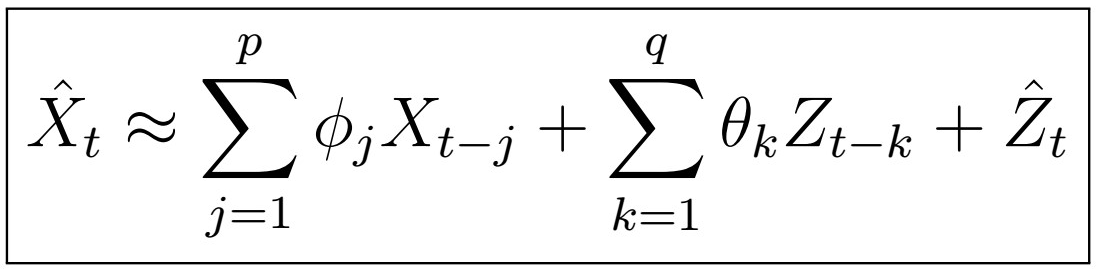# A Complete Introduction To Time Series Analysis (with R):: Estimation of ARMA(p,q) Coefficients (Part II)The ARMA(p,q) model implies that X_{t} can be expressed in the form above.

In the last article, we learned about two algorithms to estimate the AR(p) process coefficients: the Yale-Walker equations method, and Burg’s algorithm. In this article, we will now see a very simple way to determine the MA(q) process coefficients, and a first approach to estimate the ARMA(p,q), jointly. Let’s…

--

--